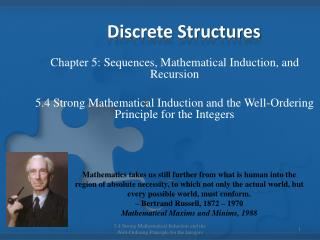Download PresentationDiscrete Structures

Loading in 2 Seconds...

# Discrete Structures - PowerPoint PPT Presentation

Discrete Structures. Chapter 5: Sequences, Mathematical Induction, and Recursion 5.4 Strong Mathematical Induction and the Well-Ordering Principle for the Integers.I am the owner, or an agent authorized to act on behalf of the owner, of the copyrighted work described.
Download Presentation## Discrete Structures

An Image/Link below is provided (as is) to download presentation

Download Policy: Content on the Website is provided to you AS IS for your information and personal use and may not be sold / licensed / shared on other websites without getting consent from its author.While downloading, if for some reason you are not able to download a presentation, the publisher may have deleted the file from their server.

- - - - - - - - - - - - - - - - - - - - - - - - - - E N D - - - - - - - - - - - - - - - - - - - - - - - - - -
Presentation Transcript
1. Discrete Structures Chapter 5: Sequences, Mathematical Induction, and Recursion 5.4 Strong Mathematical Induction and the Well-Ordering Principle for the Integers Mathematics takes us still further from what is human into the region of absolute necessity, to which not only the actual world, but every possible world, must conform. – Bertrand Russell, 1872 – 1970 Mathematical Maxims and Minims, 1988 5.4 Strong Mathematical Induction and the Well-Ordering Principle for the Integers

2. Introduction • Strong mathematical induction is similar to ordinary mathematical. • However, the basis step may contain proofs for several initial values. In the inductive step, the truth of the predicate is assumed for all values through k. 5.4 Strong Mathematical Induction and the Well-Ordering Principle for the Integers

3. Principle of Strong Mathematical Induction • Let P(n) be a property that is defined for all integers n, and let a and b be fixed integers with a b. Suppose the following two statements are true: • Basic Step: P(a), P(a+1), …, and P(b) are all true. • Inductive Step: For any integer k b, ifP(i) is true for all integers i from a through k, then P(k + 1) is true. Then the statement for all integers n a, P(n) is true. 5.4 Strong Mathematical Induction and the Well-Ordering Principle for the Integers

4. Inductive Hypothesis • The supposition that P(i) is true for all integers i from a through k is called the inductive hypothesis. • Another way to state the inductive hypothesis is to say that P(a), P(a + 1), …, P(k) are all true. 5.4 Strong Mathematical Induction and the Well-Ordering Principle for the Integers

5. Well-Ordering Principle for Integers • Let S be a set of integers containing one or more integers all of which are greater than some fixed integer. Then S has a least element 5.4 Strong Mathematical Induction and the Well-Ordering Principle for the Integers

6. Example – pg. 277 #2 5.4 Strong Mathematical Induction and the Well-Ordering Principle for the Integers

7. Example – pg. 277 #3 5.4 Strong Mathematical Induction and the Well-Ordering Principle for the Integers

8. Example – pg. 277 #15 • Any sum of two or more integers is a result of successive additions of two integers at a time. For instance, here are a few of the ways in which a1 + a2 + a3 + a4 might be computed: (a1 + a2) + (a3 + a4) OR (((a1 + a2) + a3) + a4) OR a1 + ((a2 + a3) + a4). Use strong mathematical induction to prove that any sum of two or more even integers is even. 5.4 Strong Mathematical Induction and the Well-Ordering Principle for the Integers

9. Example – pg. 277 #21 • Use the well-ordering principle for the integers to prove the existence part of the unique factorization of integers theorem: • Every integer greater than 1 is either prime or a product of prime numbers. 5.4 Strong Mathematical Induction and the Well-Ordering Principle for the Integers

10. Example – pg. 277 #24 • Use the well-ordering principle to prove that given any integer n 1, there exists an odd integer m and a nonnegative integer k such that n = 2km 5.4 Strong Mathematical Induction and the Well-Ordering Principle for the Integers